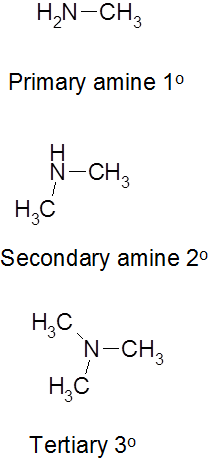# 25.1 Introduction to Amines

$$\newcommand{\vecs}{\overset { \rightharpoonup} {\mathbf{#1}} }$$ $$\newcommand{\vecd}{\overset{-\!-\!\rightharpoonup}{\vphantom{a}\smash {#1}}}$$$$\newcommand{\id}{\mathrm{id}}$$ $$\newcommand{\Span}{\mathrm{span}}$$ $$\newcommand{\kernel}{\mathrm{null}\,}$$ $$\newcommand{\range}{\mathrm{range}\,}$$ $$\newcommand{\RealPart}{\mathrm{Re}}$$ $$\newcommand{\ImaginaryPart}{\mathrm{Im}}$$ $$\newcommand{\Argument}{\mathrm{Arg}}$$ $$\newcommand{\norm}{\| #1 \|}$$ $$\newcommand{\inner}{\langle #1, #2 \rangle}$$ $$\newcommand{\Span}{\mathrm{span}}$$ $$\newcommand{\id}{\mathrm{id}}$$ $$\newcommand{\Span}{\mathrm{span}}$$ $$\newcommand{\kernel}{\mathrm{null}\,}$$ $$\newcommand{\range}{\mathrm{range}\,}$$ $$\newcommand{\RealPart}{\mathrm{Re}}$$ $$\newcommand{\ImaginaryPart}{\mathrm{Im}}$$ $$\newcommand{\Argument}{\mathrm{Arg}}$$ $$\newcommand{\norm}{\| #1 \|}$$ $$\newcommand{\inner}{\langle #1, #2 \rangle}$$ $$\newcommand{\Span}{\mathrm{span}}$$$$\newcommand{\AA}{\unicode[.8,0]{x212B}}$$

Amines are derivatives of ammonia in which one or more of the hydrogens has been replaced by an alkyl or aryl group.

## The basic properties of amines

We are going to have to use two different definitions of the term "base" in this page. A base is

• a substance which combines with hydrogen ions. This is the Bronsted-Lowry theory.
• an electron pair donor. This is the Lewis theory.

The easiest way of looking at the basic properties of amines is to think of an amine as a modified ammonia molecule. In an amine, one or more of the hydrogen atoms in ammonia has been replaced by a hydrocarbon group. Replacing the hydrogens still leaves the lone pair on the nitrogen unchanged - and it is the lone pair on the nitrogen that gives ammonia its basic properties. Amines will therefore behave much the same as ammonia in all cases where the lone pair is involved.

## The reactions of amines with acids

These are most easily considered using the Bronsted-Lowry theory of acids and bases - the base is a hydrogen ion acceptor. We'll do a straight comparison between amines and the familiar ammonia reactions. Ammonia reacts with acids to produce ammonium ions. The ammonia molecule picks up a hydrogen ion from the acid and attaches it to the lone pair on the nitrogen.If the reaction is in solution in water (using a dilute acid), the ammonia takes a hydrogen ion (a proton) from a hydroxonium ion. (Remember that hydrogen ions present in solutions of acids in water are carried on water molecules as hydroxonium ions, H3O+.)

NH3(aq)+H3O+(aq)NH+4+H2O(l)

If the acid was hydrochloric acid, for example, you would end up with a solution containing ammonium chloride - the chloride ions, of course, coming from the hydrochloric acid. You could also write this last equation as:

NH3(aq)+H+NH+4(aq)

. . . but if you do it this way, you must include the state symbols. If you write H+ on its own, it implies an unattached hydrogen ion - a proton. Such things don't exist on their own in solution in water. If the reaction is happening in the gas state, the ammonia accepts a proton directly from the hydrogen chloride:

NH3(aq)+HCl(g)NH+4(s)+Cl(s)

This time you produce clouds of white solid ammonium chloride.

The designation 1o, 2o and 3o is determined by the number of alkyl groups attached to the nitrogen.## Contributors

This page titled 25.1 Introduction to Amines is shared under a not declared license and was authored, remixed, and/or curated by Layne Morsch.